# How can electricity be detected and tested?

Shop for Voltage Testers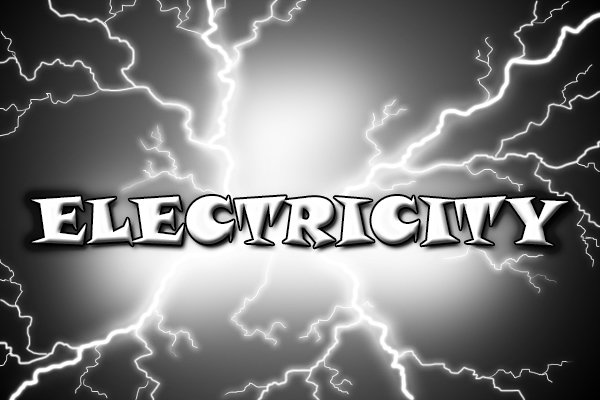There are many different components in an electrical circuit and different things to measure. Some of the tools which can measure these various things will be specific for one measurement, but many will combine measurements into one tool. Things to measure include:

## CurrentCurrent is the flow of electricity and is measured in amperes (amps, A). A device which can measure current is known as an ‘ammeter’.

To measure current, the measuring device must be inserted in series with the circuit so that the electrons pass through the ammeter at the same rate they pass through the circuit.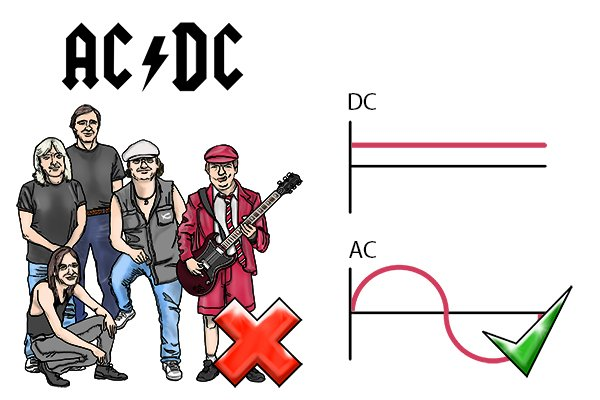Current can either be direct or alternating (DC or AC). This is due to the way in which electrons travel around a circuit, either direct; in one direction; or alternating; backwards and forwards.

## Potential difference (voltage)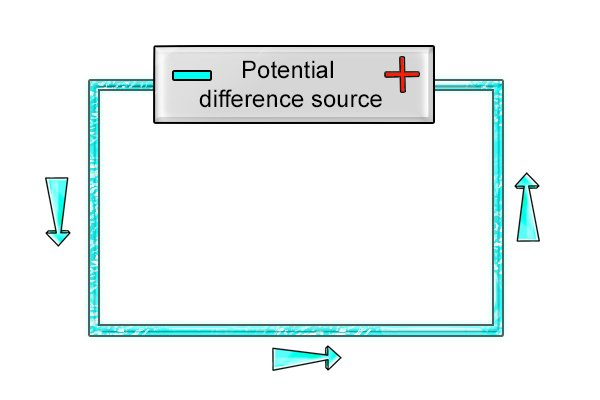Voltage is the potential difference between two points in a circuit, and is provided by what we would think of as the power source in a circuit; the battery or the plug socket (mains electricity).

In order to measure voltage, a device called a voltmeter must be inserted in parallel to the circuit.

## Resistance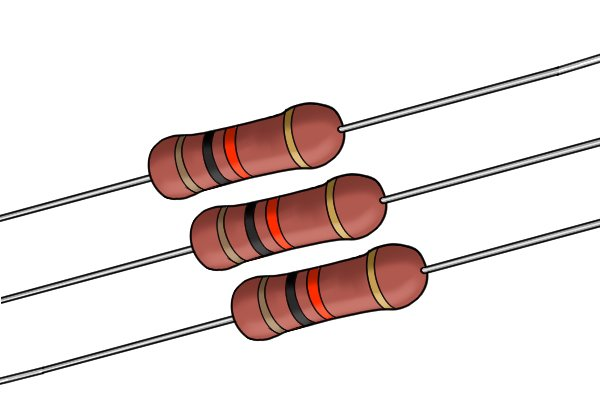Resistance is measured in ohms (Ω) and is to do with how the conductor material allows the current to pass through it.

For instance, a short cable has lower resistance than a long cable because there is less material for the current to pass through.

A device which can measure resistance is called an ‘ohmmeter’.

## Current, resistance & potential difference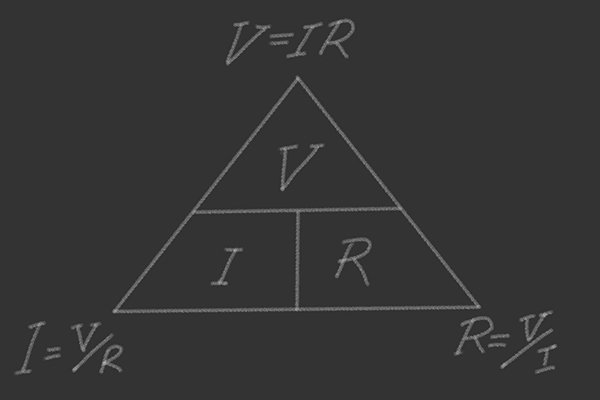There is a relationship between the volts, amps and ohms in an electrical circuit. This is known as Ohm’s law, represented by a triangle, where V is voltage, R is resistance and I is current.

The equation for this relationship is amps x ohms = volts. Therefore if you have two of the measurements, you will be able to work out the other one.

## Power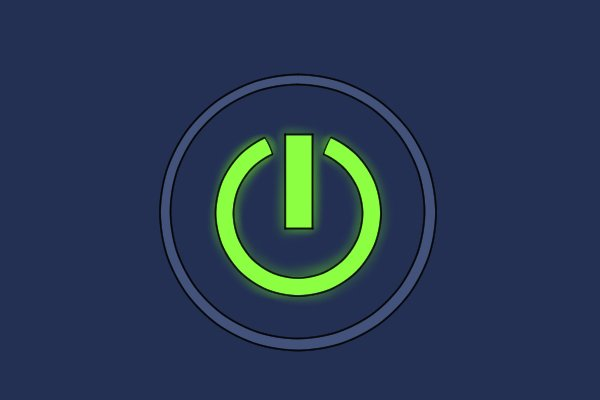Power is measured in watts (W). In electrical terms, a watt is the work done when one amp flows through one volt.

## Polarity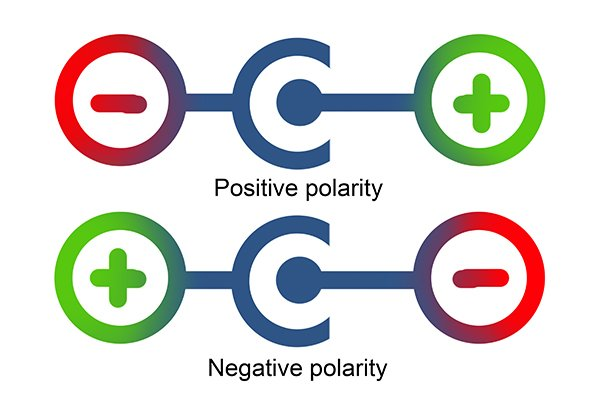Polarity is the orientation of the positive and negative points in a circuit. Polarity is technically only found in DC circuits, but because in mains electricity (AC) one wire is grounded, this creates hot (live) and neutral terminals on sockets and connections, which can be thought of as polarity.

Generally, polarity is stated on most items (like batteries) but it may be necessary to check polarity on some devices, such as speakers, where it has been missed.As identifying polarity can involve both distinguishing the positive from the negative and the hot from the neutral, there are a few different tools which can test this, including voltage detectors and multimeters.

## Continuity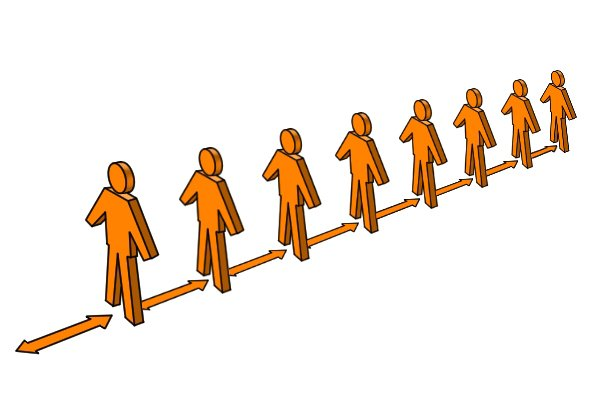Continuity is the checking of a circuit to determine if it is working or not. A continuity test tells you if electricity will be able to flow through the item you are testing or whether the circuit is somehow broken.

## Capacitance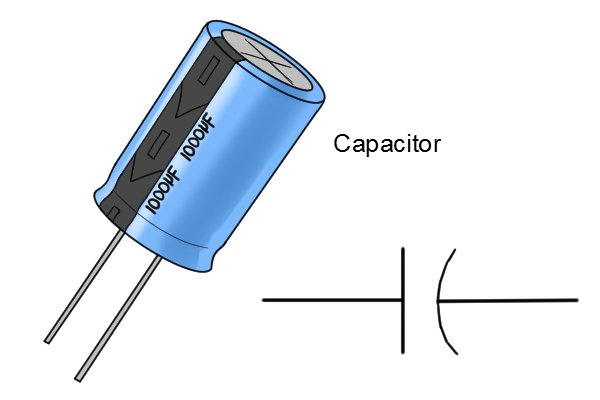Capacitance is the ability of an item to store a charge, and is measured in farads (F) or microfarads (μF). A capacitor is a component added to a circuit to store the charge.

## Frequency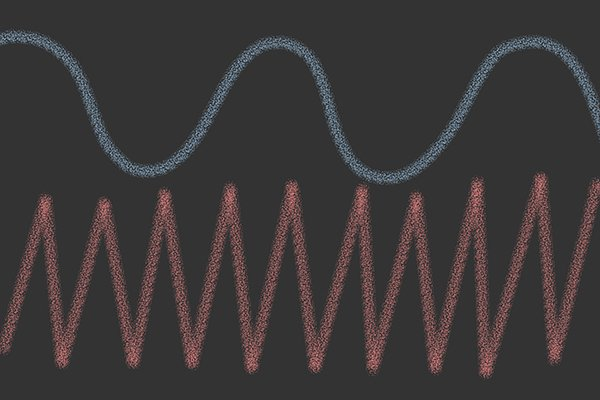Frequency is found in AC circuits and is measured in hertz (Hz). Frequency is the amount of oscillations in an AC current. That means how many times the current changes direction per unit of time.